# OliNo

Renewable Energy

## Practical measurements on a 12V DC dimmer used by OliNo

Posted by Marcel van der Steen in Explanation, Lamps
Tags: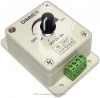When OliNo measures lamps working on 12 V DC and these lamps are dimmable, then it as a 12 V DC dimmer available to use.

This dimmer is put between the 12 V DC power supply output and the lamp itself.

It appears that this dimmer uses a pulse width modulation (PWM) to switch the voltage to the lamp between a high level and a low level; this at a speed invisible to the human eye. It is a very efficient way to adjust the average level of the voltage that is output to the lamp.

This article gives a number of measurements on the output of the dimmer to show what the dimmer does with the voltage to the lamp.

### Measurement setup

The dimmer is placed between the output of a 12 V DC power supply and the lamp that is normally fed with this 12 V DC.

By adjusting the position of the knob the output voltage towards the lamp can be adjusted. For an impression see the schematic below.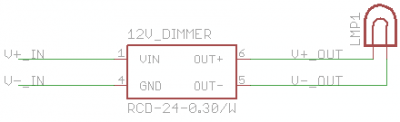Scheme of the dimmer in the line from 12 V DC output of power supply and the lamp.

### Measurement results

In this setup a led lamp has been connected to the output of the dimmer. The dimmer was connected to a 12 V DC output. The know has been set to different mechanical positions, and the V+ out and V- out at the output of the dimmer is measured and screenshots of the oscilloscope are given.

Dim position Voltage over V+ out and V- out
100 % (no dimming)

A constant voltage of 12 V DC is measured at the output of the dimmer. So the lamp is fed with a constant voltage of 12 V DC.75 % dim position.

The voltage output to the lamp varies between 8 and 12 V DC. It is about 30 % of the time at 8 V DC and the remainder of the time at 12 V DC. One cycle of 8 V and 12 V takes about 1/600th of a second, meaning that the voltage variations that result in illuminance variations of the led, will not be visible by the human eye.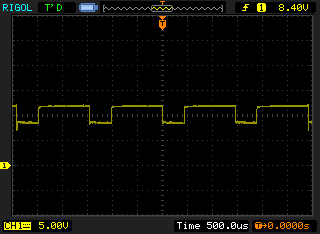50 % dim position

The voltage varies between 8 and 12 V DC. 50 % of the time at each value, every 1/600th of a second.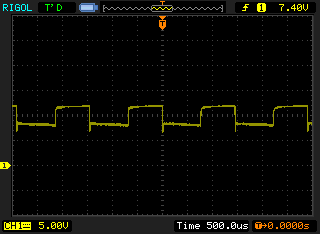25 % dim position

The voltage varies between 7 V and 12 V DC. It is 75 % of the time at 7 V and the remainder of the time at 7 V. The total cycle time of one time the 7 V and 12 V output is 1/600th f a second.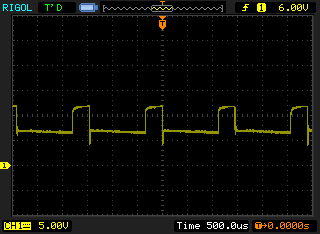12.5 % dim position

The voltage varies between -2 and 12 V DC. It is 90 % at 02 and the remainder at 12 V. The cycle time is 1/600th of a second that a cycle of -2 and 12 V is output to the lamp.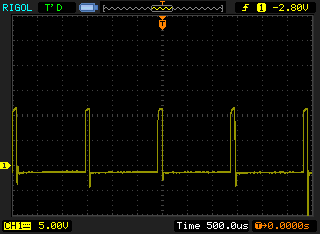8 % dim position

The voltage varies between -5 and 12 V DC> It is 95 % at -5 V and the remainder at 12 V. One cycle of -5 V and 12 V on the lamp takes 1/600th of a second.The dimmer uses pulse width modulation (PWM) and at high amount of dimming the voltage to the lamp is even getting negative. The cycletime of one cycle is 1/600th of a second which easly can result in a flickering illuminance output of the led, however it will not be visible for the human eye.

### Dim measurement results

The tested lamp is well dimmable. The voltage swings at the output of the dimmer , applied to the lamp, result in the following illuminance, power and power factor as function on the dim-position of the knob.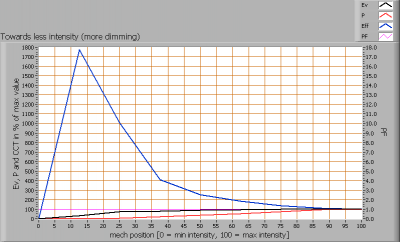Dim measurement results with the 12 V dimmer before a led lamp with MR16 base.

The results given above show that the efficacy increases a lot (normally a an led is more efficient at lower currents because of less internal heat and hence lower internal temperature). To zoom in more on the area 0 – 100 % see the image below..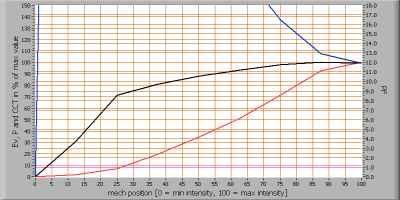Dim measurement results more in detail.

The illuminance Ev is well selectable depending on the position of the mechanical knob. The range for the illuminance Ev is from 0 – 100 %.

The power (this is the power of the lamp INCLUDING the dimmer) reduces more quickly than the reduction of the illuminance, resulting in an increased efficacy.